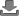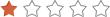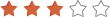clear
Keywords
clear
to
clear
Show
items
Circles, Arcs and Angles by J Pollack
Students learn about the different arcs and angles derived from circles88 - 12
21 Dec 2012
Algebra Properties by M Miller
Students learn various terms relating to algebra and apply them in examples412 - 15
21 Dec 201249 - 11
06 Aug 2013
Rounding and Naming Decimals by Cristy Holtzclaw
Students learn to name, write and round decimal numbers119 - 11
07 Dec 2012
Polygon Characteristics by Cristy Holtzclaw
Students learn to label and identify polygons, to find lines of symmetry in polygons, and to identify congruent and similar polygons57 - 11
07 Dec 2012
Fraction Wall by Edwina Jay
Recognise simple relationships between equivalent fractions447 - 11
07 Dec 2012
Introduction to Perimeter by Debbie Jones
Identify why and where perimeter is use, suitable tools and units for measuring it and students then practise measuring perimeter56 - 9
07 Dec 2012
Pattern Trains by Christine Ames
Students learn to use patterns to describe the world, solve problems and to identify, create and compare patterns arising from daily experiences in the classroom34 - 7
07 Dec 2012
Number Sequencing to 100 by Christine Ames
Students learn to use numbers to describe quantities, represent numbers in multiple ways, to recognise and apply whole numbers from 0 to 100, and to recognise, build, compare and order sets to 100105 - 7
07 Dec 2012
Ordinal Numbers to Ten by Christine Ames
Use numbers to describe quantities and represent numbers in multiple ways54 - 7
07 Dec 2012
Ten Frames by Kellie Gaffney
Understand the ones and tens positions and the numerals 10-2084 - 7
07 Dec 2012
Introduction to Probability by Louis Paul
An introduction to the concepts of probabilty using dice and cards311 - 13
07 Dec 2012
Representing numbers in base ten blocks
A workbook containing thousands, hundreds, tens and ones sets of base ten blocks for representing numbers in base ten145 - 8
07 Dec 2012
Introduction to Algebra by Cristy Holtzclaw
A brief introduction to simple algebraic variables and solving simple equations210 - 12
07 Dec 2012
Finding the Area by Jaclyn Summerlin
Students learn techniques to find the area of squares, rectangles and similar shapes, as well as design shapes for a given area85 - 10
07 Dec 2012
Perimeter
Students find the perimeter of various polygons45 - 10
07 Dec 2012
Finding the Derivative of Sine by Brian Leyhane
Students learn how to find the derivatives of 8 trigonometric functions113 - 16
07 Dec 2012
Comparing and Ordering Fractions by Brenda Ballie
Learn to order and compare fractions and appy knowledge of least common denominator to order fractions138 - 12
06 Dec 2012
The Soccer Challenge by Russell Bates
A soccer themed game for two teams. Tests basic subtraction skills135 - 10
05 Dec 2012
Pythagorean Theorem - Theory and Practise
Brief introduction to the pythagorean equation and ten practise questions complete with worked answers29 - 15
04 Dec 2012
Show
items